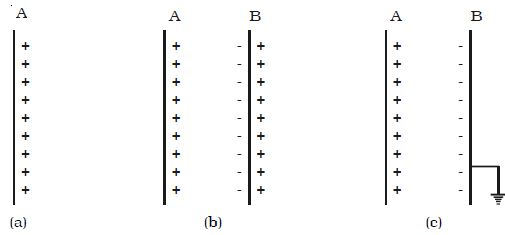# Explain Principle of a Capacitor

The principle of a CapacitorConsider an insulated conductor (Plate A) with a positive charge ‘q’ having potential V (Figure a). The capacitance of A is C = q/V.

When another insulated metal plate B is brought near A, negative charges are induced on the side of B near A. An equal amount of positive charge is induced on the other side of B (Figure b).

The negative charge in B decreases the potential of A. The positive charge in B increases the potential of A. But the negative charge on B is nearer to A than the positive charge on B. So the net effect is that, the potential of A decreases. Thus the capacitance of A is increased. If the plate B is earthed, positive charges get neutralized (Figure c). Then the potential of A decreases further. Thus the capacitance of A is considerably increased.

The capacitance depends on the geometry of the conductors and nature of the medium. A capacitor is a device for storing electric charges.Fig: Principle of the capacitor